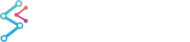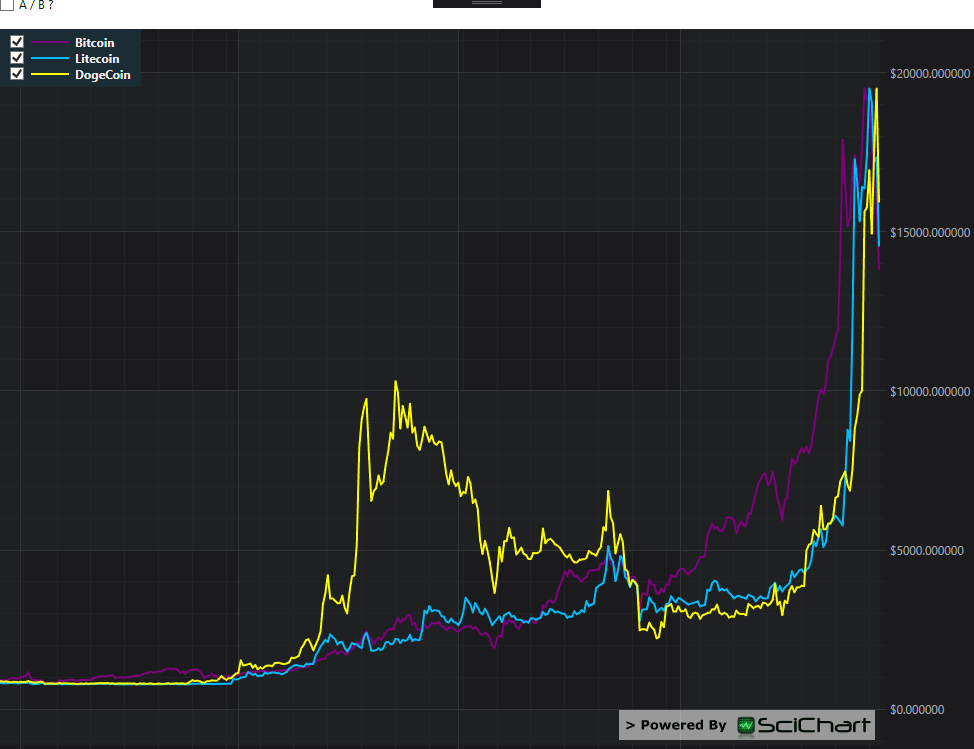﻿ Ratio Filter | WPF Chart DocumentationSciChart WPF 2D Charts > Filters API > Ratio Filter
Ratio Filter

The Ratio filter performs a vector division of two Time Series, basically dividing series A by series B.

This is useful when you want to visualize the price of asset A in terms of asset B. In the example below, we plot Bitcoin, Litecoin and DogeCoin on a SciChartSurface. At the click of a button we apply the Ratio Filter to plot Doge/BTC.

Ratio Filter
Copy Code
```// Assuming you have populated two data-series with Bitcoin and Dogecoin historical prices
_btcDataSeries = new XyDataSeries<DateTime,double>() { SeriesName = "Bitcoin" };
_dogecoinDataSeries = new XyDataSeries<DateTime, double>() { SeriesName = "DogeCoin" };

// Create the Ratio Filter. SeriesA / SeriesB
var ratioFilter = new RatioFilter<DateTime, double>(_dogecoinDataSeries, _btcDataSeries);
ratioFilter.FilteredDataSeries.SeriesName = "DogeCoin/BTC";
// Visualize as a Line Series